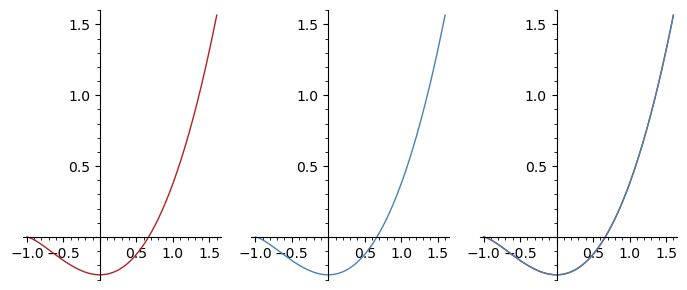# Problem by finding an integral

I have to find the integral of x*sqrt(x + 1) dx.

By hand, I found (2/3)*x*(x + 1)^(3/2) – (4/15)*(x + 1)^(5/2) + c.

By SageMath I found -(2/3)*(x + 1)^(3/2) + (2/15)*(x + 1)^(5/2).

The SageMath code is simple:

x = var('x')
f = x*sqrt(x + 1)
fi = integral(f, x)
print(latex(fi))


Which is the error?

edit retag close merge delete

Sort by » oldest newest most votedThe SageMath result (output of your code in SageMath 9.2) has coefficient a $2/5$ instead of the $2/15$ you claim:

sage: fi
2/5*(x + 1)^(5/2) - 2/3*(x + 1)^(3/2)


Then it agrees with your hand calculation, e.g. because for $z = \sqrt{x+1}$ we have $$\frac{2}{5} z^5 - \frac{2}{3} z^3= \frac{2}{3}(z^2-1)z^3 - \frac{4}{15}z^5.$$

more

You can accept rburing's answer by clicking the check mark next to it below its score.

To see it by hand, we can use:

• $(x + 1)^{5/2} = (x + 1) (x + 1)^{3/2} = x (x + 1)^{3/2} + (x + 1)^{3/2}$
• $(x + 1)^{3/2} = (x + 1) (x + 1)^{1/2} = x (x + 1)^{1/2} + (x + 1)^{1/2}$

to express both as linear combinations of $(x + 1)^{1/2}$, $x (x + 1)^{1/2}$ and $x^2 (x + 1)^{1/2}$.

To see it with Sage, we can

• ask Sage whether the two different expressions in fact agree
• differentiate both and compare them
• plot the functions to visually check whether they agree

Define the function to integrate:

sage: x = var('x')
sage: f = x*sqrt(x + 1)
sage: f
sqrt(x + 1)*x


Compute a primitive (aka antiderivative) with Sage:

sage: F = integral(f, x)
sage: F
2/5*(x + 1)^(5/2) - 2/3*(x + 1)^(3/2)


The primitive you computed (up to a constant):

sage: G = (2/3)*x*(x + 1)^(3/2) - (4/15)*(x + 1)^(5/2)
sage: G
-4/15*(x + 1)^(5/2) + 2/3*(x + 1)^(3/2)*x


Check that they agree:

sage: bool(F == G)
True


Check they have the same derivative:

sage: g = G.diff(x)
sage: g
sqrt(x + 1)*x

sage: ff = F.diff(x)
sage: ff
(x + 1)^(3/2) - sqrt(x + 1)

sage: bool(g == ff)
True


Or more visually, compare the plots of F and G (or f and ff):

sage: pF = plot(F, (-1, 1.6), color='firebrick')
sage: pG = plot(G, (-1, 1.6), color='steelblue')
sage: p = graphics_array([pF, pG, pF + pG])
sage: p.show(figsize=(7, 3))
Launched png viewer for Graphics Array of size 1 x 3more

Excellent! Very interesting answer. Otherwise, I have an older version: Sage 8.0

Oh, I see. I would recommend upgrading to Sage 9.3.

When I run Sage 8.0 I get this:

sage: x = var('x')
sage: f = x*sqrt(x + 1)
sage: f
sqrt(x + 1)*x
sage: F = integral(f, x)
sage: F
2/5*(x + 1)^(5/2) - 2/3*(x + 1)^(3/2)


Do you really get (2/15)?

Yeah, the problem probably has to do with the version. I uninstalled the 8.0 version and I installed the 9.3 one. Now everything is o.k. Thanks a lot!